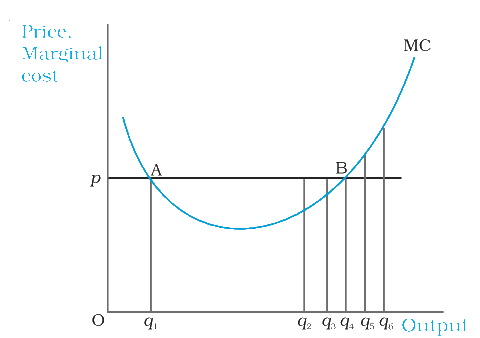# Profit Maximisation

## What is profit maximisation?

An enterprise manufactures and sells a definite amount of a commodity. The enterprise’s profit, denoted by π, is defined as the difference between its TR (total revenue) and TC (total cost of production). In other words,

π = TR – TC

The gap between TR and TC is the enterprise’s profits. An enterprise wishes to maximise its profit and likes to recognise the amount or quantity q0, at which its profits are maximum. By definition, at any quantity other than q0, the enterprise’s profits are less than q0. Here, the question is how do we recognise q0. For profits to be maximum, three conditions must hold at q0.

• The cost price p, must be equal to MC.
• The marginal cost must be non-decreasing at q0.
• For the enterprise to continue to manufacture in the short run, the cost price must be greater than the average variable cost (p > AVC), whereas in the long run, the cost price must be greater than the average cost (p > AC).## Condition 1

Profits are nothing but the difference between total revenue and total cost. Both TR and TC increase as the output increases. As long as the change in total revenue is greater than the change in total cost, the profits continue to rise. Recall that the change in TR per unit increase in the output is the marginal revenue, and the change in total cost per unit increase in the output is the marginal cost.

## Condition 2

Now, let us consider the second condition that must hold good when the profit-maximising output degree is positive. Note that at output degrees q1 and q4, the market price is equivalent to the marginal cost. However, at output level q1, the marginal cost curve is a downward graph. We claim that q1 cannot be a profit-maximising output degree.

## Condition 3

Let us consider the third condition that must hold good when the profit-maximising output degree is positive. Note that the third condition has two parts: one part is applicable in the short run while the other is applicable in the long run.